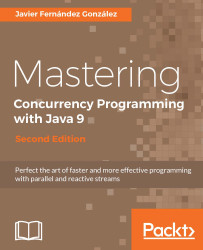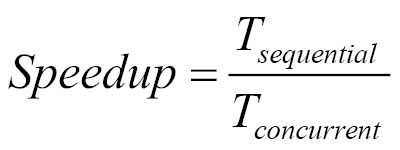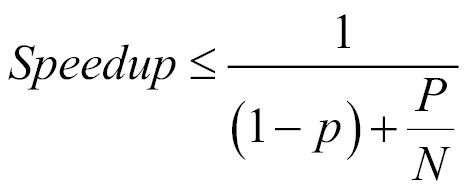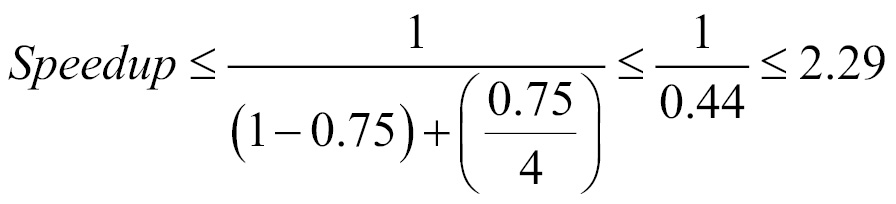•#### Mastering Concurrency Programming with Java 9 - Second Edition#### Overview of this book

Concurrency programming allows several large tasks to be divided into smaller sub-tasks, which are further processed as individual tasks that run in parallel. Java 9 includes a comprehensive API with lots of ready-to-use components for easily implementing powerful concurrency applications, but with high flexibility so you can adapt these components to your needs. The book starts with a full description of the design principles of concurrent applications and explains how to parallelize a sequential algorithm. You will then be introduced to Threads and Runnables, which are an integral part of Java 9's concurrency API. You will see how to use all the components of the Java concurrency API, from the basics to the most advanced techniques, and will implement them in powerful real-world concurrency applications. The book ends with a detailed description of the tools and techniques you can use to test a concurrent Java application, along with a brief insight into other concurrency mechanisms in JVM.
Title PageCreditswww.PacktPub.comCustomer FeedbackDedicationPrefaceFree Chapter
The First Step - Concurrency Design PrinciplesWorking with Basic Elements - Threads and RunnablesManaging Lots of Threads - ExecutorsGetting the Most from ExecutorsGetting Data from Tasks - The Callable and Future InterfacesRunning Tasks Divided into Phases - The Phaser ClassOptimizing Divide and Conquer Solutions - The Fork/Join FrameworkProcessing Massive Datasets with Parallel Streams - The Map and Reduce ModelProcessing Massive Datasets with Parallel Streams - The Map and Collect ModelAsynchronous Stream Processing - Reactive StreamsDiving into Concurrent Data Structures and Synchronization UtilitiesTesting and Monitoring Concurrent ApplicationsConcurrency in JVM - Clojure and Groovy with the Gpars Library and Scala## A methodology to design concurrent algorithms

In this section, we're going to propose a five-step methodology to get a concurrent version of a sequential algorithm. It's based on the one presented by Intel in their Threading Methodology: Principles and Practices document.

### The starting point - a sequential version of the algorithm

Our starting point to implement a concurrent algorithm will be a sequential version of the algorithm. Of course, we could design a concurrent algorithm from scratch, but I think that a sequential version of the algorithm will give us two advantages:

• We can use the sequential algorithm to test whether our concurrent algorithm generates correct results. Both algorithms must generate the same output when they receive the same input, so we can detect some problems in the concurrent version, such as data races or similar conditions.
• We can measure the throughput of both algorithms to see if the use of concurrency gives us a real improvement in the response time or in the amount of data the algorithm can process in a time.

### Step 1 - analysis

In this step, we are going to analyze the sequential version of the algorithm to look for the parts of its code that can be executed in a parallel way. We should pay special attention to those parts that are executed most of the time or that execute more code because, by implementing a concurrent version of those parts, we're going to get a greater performance improvement.

Good candidates for this process are loops, where one step is independent of the other steps, or portions of code are independent of other parts of the code (for example, an algorithm to initialize an application that opens the connections with the database, loads the configuration files, and initializes some objects; all these tasks are independent of each other).

### Step 2 - design

Once you know what parts of the code you are going to parallelize, you have to decide how to do that parallelization.

The changes in the code will affect two main parts of the application:

• The structure of the code
• The organization of the data structures

You can take two different approaches to accomplish this task:

• Task decomposition: You do task decomposition when you split the code into two or more independent tasks that can be executed at once. Maybe some of these tasks have to be executed in a given order or have to wait at the same point. You must use synchronization mechanisms to get this behavior.
• Data decomposition: You do data decomposition when you have multiple instances of the same task that work with a subset of the dataset. This dataset will be a shared resource, so if the tasks need to modify the data, you have to protect access to it, implementing a critical section.

Another important point to keep in mind is the granularity of your solution. The objective of implementing a parallel version of an algorithm is to achieve improved performance, so you should use all the available processors or cores. On the other hand, when you use a synchronization mechanism, you introduce some extra instructions that must be executed. If you split the algorithm into a lot of small tasks (fine-grained granularity), the extra code introduced by the synchronization can provoke performance degradation. If you split the algorithm into fewer tasks than cores (coarse-grained granularity), you are not taking advantage of all resources. Also, you must take into account the work every thread must do, especially if you implement a fine-grained granularity. If you have a task longer than the rest, that task will determine the execution time of the application. You have to find the equilibrium between these two points.

### Step 3 - implementation

The next step is to implement the parallel algorithm using a programming language and, if it's necessary, a thread library. In the examples of this book, you are going to use Java to implement all the algorithms.

### Step 4 - testing

After finishing the implementation, you should test the parallel algorithm. If you have a sequential version of the algorithm, you can compare the results of both algorithms to verify that your parallel implementation is correct.

Testing and debugging a parallel implementation are difficult tasks because the order of execution of the different tasks of the application is not guaranteed. In Chapter 12, Testing and Monitoring Concurrent Applications, you will learn tips, tricks, and tools to do these tasks efficiently.

### Step 5 - tuning

The last step is to compare the throughput of the parallel and the sequential algorithms. If the results are not as expected, you must review the algorithm, looking for the cause of the bad performance of the parallel algorithm.

You can also test different parameters of the algorithm (for example, granularity, or number of tasks) to find the best configuration.

There are different metrics to measure the possible performance improvement you can obtain parallelizing an algorithm. The three most popular metrics are:

• Speedup: This is a metric for relative performance improvements between the parallel and the sequential versions of the algorithm:Here, Tsequential is the execution time of the sequential version of the algorithm and Tconcurrent is the execution time of the parallel version.
• Amdahl's law: Used to calculate the maximum expected improvement obtained with the parallelization of an algorithm:Here, P is the percentage of code that can be parallelized and N is the number of cores of the computer where you're going to execute the algorithm.

For example, if you can parallelize 75% of the code and you have four cores, the maximum speedup will be given by the following formula:• Gustafson-Barsis' law: Amdahl's law has a limitation. It supposes that you have the same input dataset when you increase the number of cores, but normally, when you have more cores, you want to process more data. Gustafson's law proposes that when you have more cores available, bigger problems can be solved at the same time using the following formula:Here, N is the number of cores and P is the percentage of parallelizable code.

If we use the same example as before, the scaled speedup calculated by the Gustafson law is:### Conclusion

In this section, you learned some important issues you have to take into account when you want to parallelize a sequential algorithm.

First of all, not every algorithm can be parallelized. For example, if you have to execute a loop where the result of iteration depends on the result of the previous iteration, you can't parallelize that loop. Recurrent algorithms are another example of algorithms that can be parallelized for a similar reason.

Another important thing you have to keep in mind is that the sequential version with better performance of an algorithm can be a bad starting point to parallelize it. If you start parallelizing an algorithm and you find yourself in trouble because you cannot easily find independent portions of the code, you have to look for other versions of the algorithm and verify that the version can be parallelized in an easier way.

Finally, when you implement a concurrent application (from scratch or based on a sequential algorithm), you must take into account the following points:

• Efficiency: The parallel algorithm must end in less time than the sequential algorithm. The first goal of parallelizing an algorithm is that its running time is less than the sequential one, or it can process more data in the same time.
• Simplicity: When you implement an algorithm (parallel or not), you must keep it as simple as possible. It will be easier to implement, test, debug, and maintain, and it will have less errors.
• Portability: Your parallel algorithm should be executed on different platforms with minimum changes. As in this book you will use Java, this point will be very easy. With Java, you can execute your programs on every operating system without any changes (if you implement the program as you must).
• Scalability: What happens to your algorithm if you increase the number of cores? As mentioned before, you should use every available core so your algorithm is ready to take advantage of all available resources.